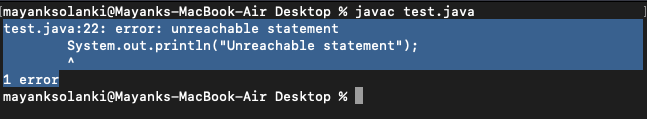GFG App
Open AppBrowser
Continue

# Unreachable statement using final and non-final variable in Java

Unreachable statements in Java refers to those sets of statements that won’t get executed during the execution of the program are called Unreachable Statements. These statements might be unreachable because of the following reasons which are as follows:

• Have a return statement before them
• Have an infinite loop before them
• Execution forcibly terminated before them

Example 1

## Java

 `// Java program to Illustrate Unreachable Statement` `// Throwing Exception` `// Using Final Variable`   `// Class 1` `// Main class` `class` `UnreachableUsingFinal {`   `    ``// Declaring and initializing final variable` `    ``final` `int` `a = ``14``, b = ``16``;`   `    ``// Method of this class` `    ``void` `compare()` `    ``{`   `        ``// Checking if number is lesser or not` `        ``while` `(a < b) {`   `            ``// First print statement` `            ``System.out.println(` `                ``"Hello i am in infinite loop"``);`   `            ``// last print statement, in this case` `            ``// unreachable` `            ``System.out.println(``"Unreachable statement"``);` `        ``}` `    ``}` `}`   `// Class 2` `// Main class` `class` `GFG {`   `    ``// Main driver method` `    ``public` `static` `void` `main(String args[])` `    ``{`   `        ``// Creating object of class 1 inside main()` `        ``UnreachableUsingFinal uuf` `            ``= ``new` `UnreachableUsingFinal();`   `        ``// Calling the method of class 1` `        ``uuf.compare();` `    ``}` `}`

Output:Output explanation:

This is because the statement ‘System.out.println(“Unreachable statement”)’,  will never be executed and java doesn’t support unreachable statements. Making those variables final also makes them constant variables and in java or any other programming language, the value of the constant variable can’t be changed. So, throughout the life cycle of the program, no condition will be approached to come out of the while loop.
This, in turn, makes the second statement unreachable i.e. JVM can’t reach that statement during the program life cycle. So, it is giving an “unreachable statement” error. Here, the program is right if looked from the coding point of view but what actually JVM thinks is that if in any condition a statement is not executed, then what is the need of using that statement? So, try to avoid this type of situation.

What if the above syso statement is commented?

Geek you must be wondering the same what if  it is commented out or removed then what will be the expected output will be fuflfiled. Yes you are right, hold and think about it as the required code will also fulfil condition of containing unreachable statement. It is as shown below in the programs as follows:

Example 2

## Java

 `// Java program to Illustrate Unreachable Statement` `// Running Into Infinite Loop ` `// Using Final Variable`   `// Class 1` `// Main class` `class` `UnreachableUsingFinal {`   `    ``// Declaring and initializing final variable` `    ``final` `int` `a = ``14``, b = ``16``;`   `    ``// Method of this class` `    ``void` `compare()` `    ``{`   `        ``// Checking if number is lesser or not` `        ``while` `(a < b) {`   `            ``// First print statement` `            ``System.out.println(` `                ``"Hello i am in infinite loop"``);` `        ``}` `    ``}` `}`   `// Class 2` `// Main class` `class` `GFG {`   `    ``// Main driver method` `    ``public` `static` `void` `main(String args[])` `    ``{`   `        ``// Creating object of class 1 inside main()` `        ``UnreachableUsingFinal uuf` `            ``= ``new` `UnreachableUsingFinal();`   `        ``// Calling the method of class 1` `        ``uuf.compare();` `    ``}` `}`

Output:

What if the final keyword in itself is removed ?

Example 3

## Java

 `// Java program to Illustrate unreachable Statement` `// Without using final variable`   `// Class 1` `// Helper class` `class` `UnreachableWithoutUsingFinal {`   `    ``// Initialisation of variable` `    ``int` `a = ``14``, b = ``16``;`   `    ``// Method of this class` `    ``void` `compare()` `    ``{`   `        ``// Checking if two numbers are equal or not` `        ``while` `(a < b) {`   `            ``// First print statement` `            ``System.out.println(` `                ``"Hello i am in infinite loop"``);` `        ``}`   `        ``// Last print statement, in this case reachable` `        ``System.out.println(``"Unreachable statement"``);` `    ``}` `}`   `// Class 2` `// Main class` `class` `GFG {`   `    ``// Main driver method` `    ``public` `static` `void` `main(String args[])` `    ``{`   `        ``// Creating object of class 1` `        ``UnreachableWithoutUsingFinal uwuf` `            ``= ``new` `UnreachableWithoutUsingFinal();`   `        ``// Calling method of class 1` `        ``uwuf.compare();` `    ``}` `}`

Output:

Output explanation:

Here, an interesting thing happens. Removing the final keyword prints the output. But in this case, also, the last print statement is unreachable.
So, it is made possible in program as the variables are declared as non-final. So, the value of these variables can be changed in the program. JVM thinks that at any point in time there is a chance that the change the value of a and b, and the last statement may be executed, so no error is coming in the second program. It means that the second program encounters the infinite loop but there is a chance(as the variables are declared non-final) that the loop may terminate and the last statement may be executed.

Note: In the second program, while executing, the output will be TLE as the while loop is not terminating.

My Personal Notes arrow_drop_up